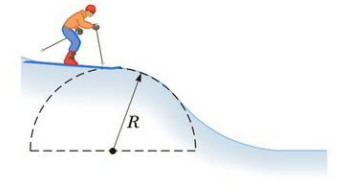Chapter 7, Problem 63AP

Chapter
Section
Textbook Problem

A skier starts at rest at the top of a large hemispherical hill (Fig. P7.63). Neglecting friction, show that the skier will leave the hill and become airborne at a distance h = R/3 below the top of the hill. Hint: At this point, the normal force goes to zero.Figure P7.63

To determine
The skier will leave the hill and will become airborne at a distance h=R/3 below the top of the hill.

Explanation

Given info: The skier starts from rest at the top of the hill. The friction is neglected.

Explanation:

The situation of the problem is shown in the following Figure 1.

Apply conservation of energy from the top if the hill till the skier comes down to a distance h below the top of the hill,

12mv2mgh=0+0

The square of the velocity at a distance h below the top of the hill will be,

v2=2gh

The radial component of the weight of the skier is toward the centre, the normal force is directed radially outward. The resultant of these will provide the centripetal acceleration. Hence,

mgcosθN=mv2R

Substitute the expression for the square of the velocity,

mgcosθN=m(2gh)R

The normal force will be,

N=mgcosθm(2gh)R

When the skier leaves the hill, the normal force will become zero

Still sussing out bartleby?

Check out a sample textbook solution.

See a sample solution

The Solution to Your Study Problems

Bartleby provides explanations to thousands of textbook problems written by our experts, many with advanced degrees!

Get Started

Name the following molecular compounds a ClF4 b CS2 c PF3 d SF6

General Chemistry - Standalone book (MindTap Course List)

What is physically exchanged during crossing over?

Human Heredity: Principles and Issues (MindTap Course List)

What is the source of alkaloids?

Chemistry for Today: General, Organic, and Biochemistry